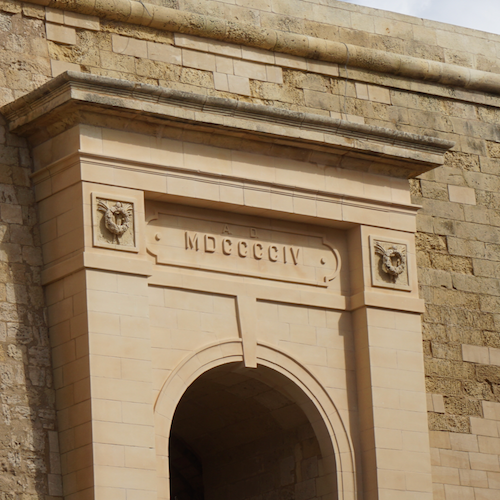# 11.13. 🤔 Converting Roman Numerals¶

I took this picture on the island of Gozo at the citadel of the city of Vittorija. The island is a small island that is part of the country of Malta.Malta has been conquered by just about everyone at one point or another in their history, including the Romans, who presumably gave us roman numerals, and example of which you can see above the arch in the picture. But, there is a problem with this one. Do you know what it is?

As I was standing there looking at it I said to my self “What kind of Roman numeral is MDCCCCIV !? You are not supposed to have four of the same letter in a row. That breaks the rules. I’m pretty sure they were trying to represent the number 1904, The question is how would you correctly represent that if you followed the rules for roman numerals?

If you are not familiar with the rules let me tell you.

1. Repeating a numeral up to three times represents the addition of the number. For example the common III is 1+1+1 = 3
2. Only I, X, C, and M can be repeated. V, L, and D should not be as there is no reason to do so. VV would simply be X and LL would be written as C
3. Writing numerals that decrease in values from left to right represents addition. For example MC represents 1000 + 100 = 1100 and XVI represents 10 + 5 + 1 or 16.
4. To write a number that would otherwise take repeating of a numeral 4 or more times there is a negation rule. Writing a smaller numeral to the left of a larger numeral causes the sign of then number on the left to be negative. for example IV is -1 + 5 = 4. Or MCMXIV is 1000 - 100 + 1000 + 10 - 1 + 5 or 1914.

Knowing all of that you can now re-write 1904 in a proper way.

DCCX-1: The correct roman numeral for 1904 is it is not MDCCCCIV.

DCCX-2: Match the roman numerals to the correct arabic numbers Make sure you follow all the rules.
• MCD
• 1400
• MCMXCVIII
• 1998
• MCMXCIX
• 1999
• MMDXLIX
• 2549
• MMCCCXLV
• 2345

## 11.13.1. Converting Roman to Arabic¶

Now that you are an expert in converting roman numerals to numbers its time to save yourself the trouble and write a program that does it for you. Here’s an outline of what you should do.

1. Ask the user to enter a roman numeral
2. Validate the number to make sure it follows all the rules. The number in the picture should cause you to print an error message.
3. If the roman numeral is valid then you should convert it to its Arabic equivalent.
4. Print out the roman numeral and its Arabic equivalent.

Hint: You will want to start by using a dictionary to map each single character roman numeral to the correct Arabic number.

## 11.13.2. Converting Arabic To Roman¶

Can you write a program to go the opposite direction? Given an Arabic number can you convert it to a roman numeral? This program uses a special kind of dictionary that preserves the order so that if you iterate over the keys you know you are going to get them in the same order you created them. (This is the default behavior for dictionaries in Python 3.6 and later, but not for our browser Python)

DCCX-3: Can you explain why the dictionary used for this part uses the keys and values it does? Why does it need to be ordered? Why does this dictionary have more entries in it than the dictionary you used for part 1?

Super Challenge:

1. Write a program that converts an incorrectly repeated number like CCCC into the proper form of CD
2. Write a program that converts a correctly negated roman numeral into a non-negated incorrect equivalent. For example IV becomes IIII
3. If you can do the first two parts then you are ready to do addition of roman numerals WITHOUT converting them to Arabic first! This one might take some extra research beyond what we have covered in class up to this point.

1. Convert any negated prefixes to additive suffixes. So, for example, IX would be rewritten to VIIII.
2. Concatenate the two strings you want to add.
3. Sort the letters, large to small. (research required)

If you’ve made it this far you have an answer but its not the simplest answer. Keep going.

1. Do internal sums (e.g., replace “IIIII” with “V”)
2. Convert back to subtractive prefixes. (e.g. replace “XIIII” with “XIV”)

Post Project Questions

During this project I was primarily in my...
• Comfort Zone
• Learning Zone
• Panic Zone
Completing this project took...
• Very little time
• A reasonable amount of time
• More time than is reasonable
Based on my own interests and needs, the things taught in this project...
• Don't seem worth learning
• May be worth learning
• Are definitely worth learning
For me to master the things taught in this project feels...
• Definitely within reach
• Within reach if I try my hardest
• Out of reach no matter how hard I try
Next Section - 11.14. 🤔 Stack Overflow Developer Survey